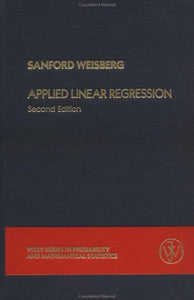Wiley

# Applied Linear Regression (Wiley Series In Probability And Statistics)

• Publish Date: 1985-07-29
• Binding: Hardcover
• Author: Sanford Weisberg

Regular price \$15.38 Sale price \$63.15

Attention: For textbook, access codes and supplements are not guaranteed with used items.

Nonlinear Statistical Methods A. Ronald Gallant Describes the recent advances in statistical and probability theory that have removed obstacles to an adequate theory of estimation and inference for nonlinear models. Thoroughly explains theory, methods, computations, and applications. Covers the three major categories of statistical models that relate dependent variables to explanatory variables: univariate regression models, multivariate regression models, and simultaneous equations models. Includes many figures which illustrate computations with SAS(R) code and resulting output. 1987 (0 471-80260-3) 610 pp. Exploring Data Tables, Trends, and Shapes Edited by David C. Hoaglin, Frederick Mosteller, and John W. Tukey Together with its companion volume, Understanding Robust and Exploratory Data Analysis, this work provides a definitive account of exploratory and robust/resistant statistics. It presents a variety of more advanced techniques and extensions of basic exploratory tools, explains why these further developments are valuable, and provides insight into how and why they were invented. In addition to illustrating these techniques, the book traces aspects of their development from classical statistical theory. 1985 (0 471-09776-4) 672 pp. Robust Regression & Outlier Detection Peter J. Rousseeuw and Annick M. Leroy An introduction to robust statistical techniques that have been developed to isolate or identify outliers. Emphasizes simple, intuitive ideas and their application in actual use. No prior knowledge of the field is required. Discusses robustness in regression, simple regression, robust multiple regression, the special case of one-dimensional location, and outlier diagnostics. Also presents an outlook of robustness in related fields such as time series analysis. Emphasizes high-breakdown methods that can cope with a sizable fraction of contamination. Focuses on the least median of squares method, which appeals to the intuition and is easy to use. 1987 (0 471-85233-3) 329 pp.